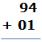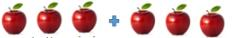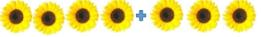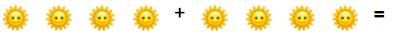Courses

## 10 Questions MCQ Test Mathematics (Maths) for Class 1 | Test: Addition - 1

Description
This mock test of Test: Addition - 1 for Class 1 helps you for every Class 1 entrance exam. This contains 10 Multiple Choice Questions for Class 1 Test: Addition - 1 (mcq) to study with solutions a complete question bank. The solved questions answers in this Test: Addition - 1 quiz give you a good mix of easy questions and tough questions. Class 1 students definitely take this Test: Addition - 1 exercise for a better result in the exam. You can find other Test: Addition - 1 extra questions, long questions & short questions for Class 1 on EduRev as well by searching above.
QUESTION: 1

Solution:
QUESTION: 2

Solution:
QUESTION: 3

### Add : 3 + 7 = ______

Solution:
QUESTION: 4

Add the following and identify the sum from the following options.Solution:
QUESTION: 5Solution:
QUESTION: 6Solution:
QUESTION: 7

Which of the following is the correct sum for the following picture?Solution:
QUESTION: 8

Write the missing numerals : 4 + _____ = 8

Solution:
QUESTION: 9

Write the missing numerals : 2 + ____ = 7

Solution:
QUESTION: 10

Which of the following is the sum of 3+4?

Solution: